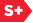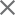### Proceedings Paper

Adaptive pseudowavelet algorithms for solving nonlinear partial differential equations
Author(s): James M. Keiser; Gregory Beylkin
Format Member Price Non-Member Price
PDF \$17.00 \$21.00

Paper Abstract

We numerically solve nonlinear partial differential equations of the form ut equals Lu + Nf(u) where L nd N are linear differential operators and f(u) is a nonlinear function. Equations of this form arise in the mathematical description of a number of phenomena including, for example, signal processing schemes based on solving partial differential equations or integral equations, fluid dynamical problems, and general combustion problems. A generic feature of the solutions of these problems is that they can possess smooth, non-oscillatory and/or shock-like behavior. In our approach we project the solution u(x,t) and the operators L and N into a wavelet basis. The vanishing moments of the basis functions permit a sparse representation of both the solution and operators, which has led us to develop fast, adaptive algorithms for applying operators to functions, e.g. Lu, and computing functions, e.g. f(u) equals u2, in the wavelet basis. These algorithms use the fact that wavelet expansions may be viewed as a localized Fourier analysis with multiresolution structure that is automatically adaptive to both smooth and shock-like behavior of the solution. In smooth regions few wavelet coefficients are needed and in singular regions large variations in the solution require more wavelet coefficients. Our new approach allows us to combine many of the desirable features of finite-difference, (pseudo) spectral and front-tracking or adaptive grid methods into a collection of efficient, generic algorithms. It is for this reason that we term our algorithms as adaptive pseudo- wavelet algorithms. We have applied our approach to a number of example problems and present numerical results.

Paper Details

Date Published: 23 October 1996
PDF: 16 pages
Proc. SPIE 2825, Wavelet Applications in Signal and Image Processing IV, (23 October 1996); doi: 10.1117/12.255269
Show Author Affiliations
James M. Keiser, Univ. of Colorado/Boulder (United States)
Gregory Beylkin, Univ. of Colorado/Boulder (United States)

Published in SPIE Proceedings Vol. 2825:
Wavelet Applications in Signal and Image Processing IV
Michael A. Unser; Akram Aldroubi; Andrew F. Laine, Editor(s)